Post

# C# Artificial Intelligence (AI) Programming: A Basic Object Oriented (OOP) Framework for Neural Networks

• 684.7k
• 0
• 21

A Neural Network is an Artificial Intelligence (AI) methodology that attempts to mimic the behavior of the neurons in our brains.  Neural networks really shine when it comes to pattern recognition and are used in image and character recognition programs, data filtering applications, and even robotics. A neural net was even used to drive an automated vehicle across the US after learning from observing human drivers.  In this article, we'll be building a basic framework for AI Neural Networks in C# and teach our program to perform basic X-OR operations.

Part I. Overview

Basically, each neuron in our brain accepts input from many other neurons and then provides a resulting output.  This is precisely what we will be replicating in code.  Each neuron class will have a structure similar to diagram 1 where there is a body of attributes and one output.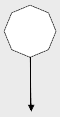Diagram 1

Each neuron can have multiple inputs and the neurons will be grouped as in diagram 2.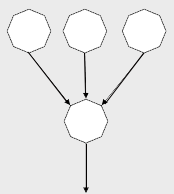Diagram 2

Neurons will be grouped in layers. While processing a signal (we'll call it a "pulse"), the signal will start at the top layer flowing through and being modified by each neuron in that layer.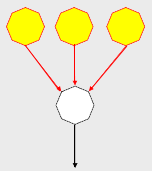Diagram 3.

Each neuron will modify the strength of the pulse.  After the modification has been completed, the "pulse" will travel to the next layer and be modified again.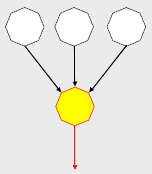Diagram 4

Now that you have the details, let's take a step back and see how a large number of cells create a "neural net", or a network of neurons. For a neural net to work, we need at least three groupings of neurons.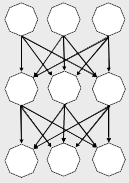Diagram 5

The top layer is used by the neural net to perceive the environment and is often called the "perception" or "input" layer.  This is where we will set initial values to be passed through the net with the pulse.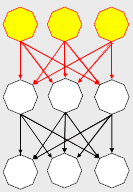Diagram 6

The bottom later is where the neural net will expose the final output of our pulse. Notice these neurons don't send their signal anywhere.  After the pulse travels to this layer, we'll go pick up the values on the output neurons as the final output of our network's processing.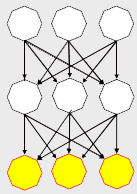Diagram 7.

Last but not least, all the neurons in the middle layer(s) process the pulse as it travels through the net but are not exposed as direct input or output of the net.  This is often called the "hidden" layer.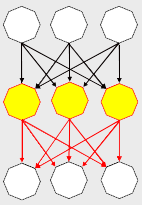Diagram 8

Part 2. Learning through back propagation.

So now for the magic: making the network learn.

In order for our neural net to have the ability to learn, after our signal travels from the top of our net to the bottom, we have to update how each neuron will affect the next pulse that travels the network.  This is done by a process called back propagation.  Basically we figure out a figure representing the level of error that our network produced.  This is arrived at by comparing the expected output of the net to the actual output.

Let's say we have an error in one of the cells in the output layer.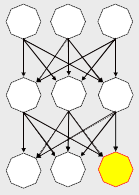Diagram 9.

Each neuron will keep track of the neurons sending the pulse through and adjust the importance of the output of each of these parent neurons that contributed to the final output of the error cell.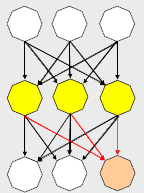Diagram 10.

Next, each of these neurons will have an error value calculated, and the adjustment will "back propagate", meaning that we will perform the same process to the next layer of neurons (the ones that send the pulse to our hidden layer).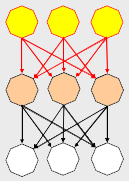Diagram 11

Conceptually, this is how the neural network will work.  Once of the cool things about neural networks is that after they learn through this iterative process and are fully trained, they can calculate output for input they have never encountered before which makes them ideal for pattern recognition and gaming AI.  Next, we'll start looking at some actual C# interfaces to represent a neural network.

Part III. The Interfaces

First we will build the basic interfaces and then implement them.  In developing scalable code, our interfaces can be the most crucial part of the project because they determine how the implementation will fall into place and ultimately the success of our project.

First, we need an interface to define signals traveling through the neurons of our network.

public interface INeuronSignal

{

double Output { get; set; }

}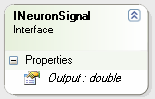Diagram 12

And we need an interface to define the input of a neuron, which is composed of the output from many other neurons.  For this, we'll use a generic dictionary where the key is a signal and the output is a class defining the "weight" of that signal.

public interface INeuronReceptor

{

Dictionary<INeuronSignal, NeuralFactor> Input { get; }

}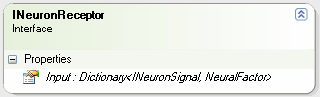Diagram 13

We could have used a double to represent the weight of each INeuronSignal in the INeuronReceptor, but we are going to be performing a technique called batch updating after a number of back propagations which will help our network learn more efficiently.  As a result, we need a class to store not only the weight of the signal, but also the amount of adjustment we'll be applying when updating.

Our NeuralFactor class will keep track of the weight and change of a neuron's input.  Even though this is not an interface, it is a part of our core AI neural net framework so I'm including it here.

public class NeuralFactor

{

#region Constructors

public NeuralFactor(double weight)

{

m_weight = weight;

m_delta = 0;

}

#endregion

#region Member Variables

private double m_weight;

private double m_delta;

#endregion

#region Properties

public double Weight

{

get { return m_weight; }

set { m_weight = value; }

}

public double Delta

{

get { return m_delta; }

set { m_delta = value; }

}

#endregion

#region Methods

public void ApplyDelta()

{

m_weight += m_delta;

m_delta = 0;

}

#endregion

}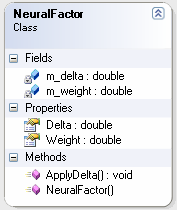Diagram 14

Next, let's define an interface for the actual neuron.  Each neuron is a receptor as well as a signal, so any object implementing our neuron interface will also implement both the INeuronSignal and INeuronReceptor interfaces.  In addition, each neuron will have a bias (think of it as another input, but one that is self-contained and not from another neuron).  This bias will have a weight just as each input to our neuron has a weight.  We'll also have methods to process a pulse and apply neuron learning.

public interface INeuron : INeuronSignal, INeuronReceptor

{

void Pulse(INeuralLayer layer);

void ApplyLearning(INeuralLayer layer);

NeuralFactor Bias { get; set; }

double BiasWeight { get; set; }

double Error { get; set; }

}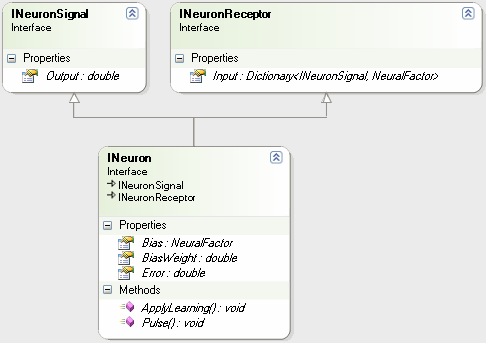Diagram 15.

Next, we'll define an interface for a layer of neurons in our neural net.  Basically, this will be used to pass the pulse or apply learning commands to each neuron in the layer.

public interface INeuralLayer : IList<INeuron>

{

void Pulse(INeuralNet net);

void ApplyLearning(INeuralNet net);

}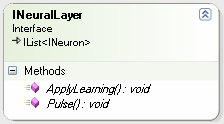Diagram 16

And our final interface will be used to define the neural net itself.  For this interface, we need to keep track of our three layers and also need to be able to pulse or apply learning to the entire neural net (passing the command to each layer which in turn passes the command to each neuron in the layer)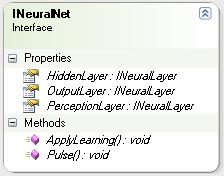Diagram 17

Part IV. Implementation Classes

Still with me?  I hope so, because now we start getting to the fun part: implementing the neural net.

1) The Neuron.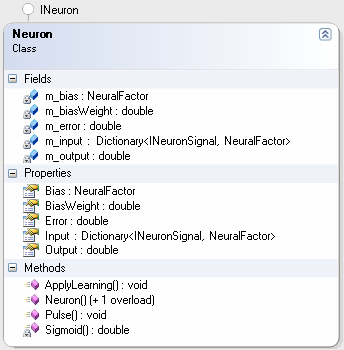Diagram 18.

The neuron has member variables used in implementing the interfaces.  The two interesting things to highlight are the Sigmoid() static function and the Pulse() method.

The Sigmoid() function uses a Sigmoid curve to squash the output of the neuron to values between 0 and 1.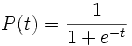private static double Sigmoid(double value)

{

return 1 / (1 + Math.Exp(-value));

}

The Pulse() method takes the sum of the value of each input (or the output of each neuron passing information to this neuron) multiplied by the respective weight contained in our dictionary.  Then adds the bias multiplied by the bias weight.  The final output is "squashed" by the sigmoid curve discussed earlier and the result is stored in the m_output variable.

Note: This means our entire network runs with values x where 0 < x < 1, or alternatively x: (0,1) (x is between 0 and 1).

public void Pulse(INeuralLayer layer)

{

lock (this)

{

m_output = 0;

foreach (KeyValuePair<INeuronSignal, NeuralFactor> item in m_input)

m_output += item.Key.Output * item.Value.Weight;

m_output += m_bias.Weight * BiasWeight;

m_output = Sigmoid(m_output);

}

}

B) The Neural Layer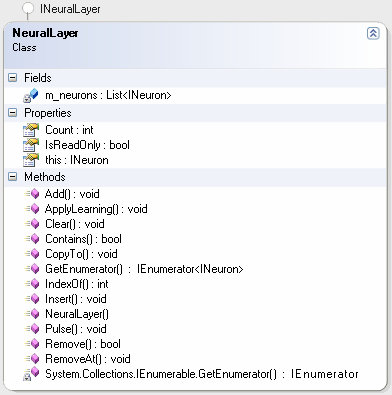Diagram 19

The NeuralLayer class is basically a collection of neurons responsible for passing a Pulse() or ApplyLearning() command through to it's member neurons.  This is implemented by wrapping a List<INeuron> and passing the IList<INeuron> methods and properties through.  The only implementation we really worry about are the following two methods:

public void Pulse(INeuralNet net)

{

foreach (INeuron n in m_neurons)

n.Pulse(this);

}

public void ApplyLearning(INeuralNet net)

{

foreach (INeuron n in m_neurons)

n.ApplyLearning(this);

}

C) The NeuralNet

Last, but definitely not least, is the NeuralNetDiagram 20

The most interesting things to note in the NeuralNet class are the m_learningRate member variable, the Train() methods, the BackPropogation() method, and the Initialize() method.

To start, let's look at the initialization of our NeuralNet.  We need to know a seed for the random number generator to construct our Neurons' NeuralFactors.  We also need to know the numbers of input neurons, hidden neurons, and output neurons.  Initialize() is our factory method and is responsible for building all of the components of our neural net and wiring them up.

public void Initialize(int randomSeed,

int inputNeuronCount, int hiddenNeuronCount, int outputNeuronCount)

{

int i, j, k, layerCount;

Random rand;

INeuralLayer layer;

// initializations

rand = new Random(randomSeed);

m_inputLayer = new NeuralLayer();

m_outputLayer = new NeuralLayer();

m_hiddenLayer = new NeuralLayer();

for (i = 0; i < inputNeuronCount; i++)

for (i = 0; i < outputNeuronCount; i++)

for (i = 0; i < hiddenNeuronCount; i++)

// wire-up input layer to hidden layer

for (i = 0; i < m_hiddenLayer.Count; i++)

for (j = 0; j < m_inputLayer.Count; j++)

new NeuralFactor( rand.NextDouble()));

// wire-up output layer to hidden layer

for (i = 0; i < m_outputLayer.Count; i++)

for (j = 0; j < m_hiddenLayer.Count; j++)

new NeuralFactor(rand.NextDouble()));

}

Next, let's look at the Pulse() and ApplyLearning() so you can see they just pass the commands to each layer, which in turn pass them to the neurons.

public void Pulse()

{

lock (this)

{

m_hiddenLayer.Pulse(this);

m_outputLayer.Pulse(this);

}

}

public void ApplyLearning()

{

lock (this)

{

m_hiddenLayer.ApplyLearning(this);

m_outputLayer.ApplyLearning(this);

}

}

Ok, now we get into the meat of the network, the BackPropogation() method.  This is where the bulk of the processing takes place. First, we calculate the errors on the output neurons by calculating the difference between what we expected (which is passed in as a parameter) and the actual output of the neuron.  After all the output neurons are updated, we calculate the errors on the hidden layer neurons in the same way.  Finally we update the adjusted weight of each neuron's inputs as well as the bias multiplied by the m_learningRate parameter.

private void BackPropogation(double[] desiredResults)

{

int i, j;

double temp, error;

INeuron outputNode, inputNode, hiddenNode, node, node2;

// Calcualte output error values

for (i = 0; i < m_outputLayer.Count; i++)

{

temp = m_outputLayer[i].Output;

m_outputLayer[i].Error = (desiredResults[i] - temp) * temp * (1.0F - temp);

}

// calculate hidden layer error values

for (i = 0; i < m_hiddenLayer.Count; i++)

{

node = m_hiddenLayer[i];

error = 0;

for (j = 0; j < m_outputLayer.Count; j++)

{

outputNode = m_outputLayer[j];

error += outputNode.Error * outputNode.Input[node].Weight * node.Output * (1.0 - node.Output);

}

node.Error = error;

}

// adjust output layer weight change

for (i = 0; i < m_hiddenLayer.Count; i++)

{

node = m_hiddenLayer[i];

for (j = 0; j < m_outputLayer.Count; j++)

{

outputNode = m_outputLayer[j];

outputNode.Input[node].Weight += m_learningRate * m_outputLayer[j].Error * node.Output;

outputNode.Bias.Delta += m_learningRate * m_outputLayer[j].Error * outputNode.Bias.Weight;

}

}

// adjust hidden layer weight change

for (i = 0; i < m_inputLayer.Count; i++)

{

inputNode = m_inputLayer[i];

for (j = 0; j < m_hiddenLayer.Count; j++)

{

hiddenNode = m_hiddenLayer[j];

hiddenNode.Input[inputNode].Weight += m_learningRate * hiddenNode.Error * inputNode.Output;

hiddenNode.Bias.Delta += m_learningRate * hiddenNode.Error * inputNode.Bias.Weight;

}

}
}

After BackPropogation, we are prepared to apply the lessons learned by comparing the actual vs. expected output on our neural network.  Doing this in batches helps out network to not over-compensate for errors occurring with different inputs.  When we are ready, we just call ApplyLearning() and our neural net will be updated.

The Train() methods, just applies an input, pulses the net, and performs the back propagation necessary for learning.

public void Train(double[] input, double[] desiredResult)

{

int i;

if (input.Length != m_inputLayer.Count)

throw new ArgumentException(string.Format("Expecting {0} inputs for this net", m_inputLayer.Count));

// initialize data

for (i = 0; i < m_inputLayer.Count; i++)

{

Neuron n = m_inputLayer[i] as Neuron;

if (null != n) // maybe make interface get;set;

n.Output = input[i];

}

Pulse();

BackPropogation(desiredResult);

}

public void Train(double[][] inputs, double[][] expected)

{

for (int i = 0; i < inputs.Length; i++)

Train(inputs[i], expected[i]);

}

So our four simple steps for neural net learning are as follows:

Step 1: Set input data into perception layer
Step 2: Pulse()
Step 3: BackPropogate()
Step 4: ApplyLearning()

Part V. Actually Doing Something -- XOR

We want to train a neural net to perform an XOR operation on two bits.  We'll build a neural net with two input neurons, two hidden neurons, and one output neuron. We want to train the net to perform the following operation.

 Input A Input B XOR Output 0 0 0 0 1 1 1 0 1 1 1 0

The problem is that we are dealing with fuzzy Boolean numbers.  Our entire neural net runs with the double data type having values between 0 and 1 and we need to get crisp values out of the net.

Not to worry, we just have to fuzzify our input and defuzzify out output.  For the inputs, instead of using the value 1, we'll use a "big" number (like 0.9) and for the value 0 we'll substitute a "small" number (like 0.1).  We'll use these same values for expected values during training.  After training, for our output, we'll say anything 0.5 and above is a 1 and anything below 0.5 is a 0.

We'll create a button for training our neural net, initialize it, and run through iterations of 100 training sessions for each application of learning.  It would be interesting to see how many training passes are required to get our net up to speed, so we'll count the number of iterations required.

private void button1_Click(object sender, EventArgs e)

{

net = new NeuralNet();

double high, mid, low;

high = .9;

low = .1;

mid = .5;

// initialize with

//   2 perception neurons

//   2 hidden layer neurons

//   1 output neuron

net.Initialize(1, 2, 2, 1);

double[][] input = new double[];

input = new double[] {high, high};

input = new double[] {low, high};

input = new double[] {high, low};

input = new double[] {low, low};

double[][] output = new double[];

output = new double[] { low };

output = new double[] { high };

output = new double[] { high };

output = new double[] { low };

double ll, lh, hl, hh;

int count;

count = 0;

do

{

count++;

for (int i = 0; i < 100; i++)

net.Train(input, output);

net.ApplyLearning();

net.PerceptionLayer.Output = low;

net.PerceptionLayer.Output = low;

net.Pulse();

ll = net.OutputLayer.Output;

net.PerceptionLayer.Output = high;

net.PerceptionLayer.Output = low;

net.Pulse();

hl = net.OutputLayer.Output;

net.PerceptionLayer.Output = low;

net.PerceptionLayer.Output = high;

net.Pulse();

lh = net.OutputLayer.Output;

net.PerceptionLayer.Output = high;

net.PerceptionLayer.Output = high;

net.Pulse();

hh = net.OutputLayer.Output;

}

while (hh > mid || lh < mid || hl < mid || ll > mid);

MessageBox.Show((count*100).ToString() + " iterations required for training");

}

Now that training has been completed, we can use the net as a tool to perform our xor operations (take a look at the complete article code).

Part V. Conclusion.

On a final note, another application (often used in gaming AI) consists of having multiple output neurons each with an associated action. After observation of the environment and pulsing the network, the node with the highest output value is determined to be the "winner" and the associated action is taken.  This is called the "winner take all" approach.

I hope you enjoyed this article.  It is meant to an introduction as there are many aspects to neural net programming that we did not get into. Efficient training of neural net is a huge subject to cover by itself. But I imagine you are pretty beat by this time...

Until next time--

Recommended Free Ebook
Similar Articles